A Fixed Point Theorem for Left Amenable Semi-Topological Semi Groups | OMICS International
Journal of Physical Mathematics

# A Fixed Point Theorem for Left Amenable Semi-Topological Semi Groups

Department of Mathematics, Tarbiat Modares University, Iran

*Corresponding Author:
Department of Mathematics
Tarbiat Modares University, Iran
Tel: 00989127309428
E-mail: [email protected]

Received Date: April 12, 2015 Accepted Date: May 14, 2015 Published Date: May 22, 2015

Citation: Naderi F (2015) A Fixed Point Theorem for Left Amenable Semi-Topological Semi Groups. J Phys Math 6:138. doi:10.4172/2090-0902.1000138

Visit for more related articles at Journal of Physical Mathematics

#### Abstract

In this note, we extend and improve the corresponding result of Takahashi. Explanation of DeMarr’s theorem is
further generalized for some semi groups of non-expansive self- maps on K by the following considerations which are explained in the paper. The application of Zorn’s lemma and its application are explained. An application of Zorn’s lemma shows that there exists a minimal non-empty compact convex and S-invariant subset.

#### Keywords

Non-expansive mappings; Semi-topological semi groups; Amenable; Left reversible

#### Introduction

Let K be a subset of a Banach space E. A self-mapping T on K is said to be non- expansive if ||T (x) -T (y)|| ≤ ||x- y|| for all x, y ∈ K. In  DeMarr proved the following theorem:

Theorem 1.1: For any non-empty compact convex subset K of a Banach space E, each commuting family of non-expansive selfmappings on K has a common fixed point in K.

DeMarr’s theorem can be further generalized for some semigroups of non-expansive self- maps on K by the following considerations.

Let S be a semi-topological semigroup, i.e. S is a semigroup with a Hausdorff topology such that for each a ∈ S, the mappings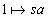and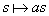from S into S are continuous. S is called left reversible if any two closed right ideals of S have non-void intersection. Let l(S) be the C*-algebra of all bounded complex-valued functions on S with supremum norm and point-wise multiplication. For each s ∈ S and f ∈ l(S), denote by ls(f) and rs(f) the left and right translates of f by s respectively, that is lsf(t)=f (st) and rsf (t)=f (ts) for all t ∈ S. Let X be a closed subspace of l(S) containing constants and be invariant under translations. Then a linear functional m ∈ X * is called a mean if ||m||=m(1)=1, and a left invariant mean (LIM) if moreover m(ls(f))=m(f) for s ∈ S, f ∈ X. Let Cb(S) be the space of all bounded continuous complex-valued functions on S with supremum norm and LUC (S) be the space of left uniformly continuous functions on S, i.e., all functions f ∈ Cb(S) for which the mapping s → lsf : S → Cb(S) is continuous when Cb(S) has the sup-norm topology. Then LUC(S) is a C *-subalgebra of Cb(S) invariant under translations and containing constant functions. S is called left amenable if LUC (S) has a LIM. The space of all right uniformly continuous functions, RUC(S), and right amenability are defined similarly. The semi-topological semigroup S is called amenable if it is both left and right amenable, in this situation there is a mean which is both left and right invariant. Left amenable semi-topological semigroups include commutative semigroups, as well as compact and solvable groups. The free (semi)group on two or more generators is not left amenable. When S is discrete, LUC(S)=l(S) and (left) amenability of S yields the (left) reversibility of S. For more details on amenability, examples and relations [2-5].

An action of S on a topological space E is a mapping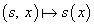from S × E into E such that (st)(x)=s(t(x)) for s, t ∈ S, x ∈ E. The action is separately continuous if it is continuous in each variable when the other is kept fixed. Every action of S on E induces a representation of S as a semigroup of self-mappings on E denoted by S, and the two semigroups are usually identified. When the action is separately continuous, each member of S is a continuous mapping on E. A subset K ⊆ E is called S-invariant if sK ⊆ K for each s ∈ S. We say that S has a common fixed point in E, if there exists a singleton S-invariant subset of E. When E is a normed space the action of S on E is called nonexpansive if ||s(x) − s(y)|| ≤ ||x – y|| for all s ∈ S and x, y ∈ E.

Takahashi  proved a generalization of DeMarr’s fixed point theorem as follows:

Theorem 1.2: Let K be a non-empty compact convex subset of a Banach space E and S be an amenable discrete semigroup which acts on K separately continuous and non-expansive. Then S has a common fixed point in K. It is well-known that every left amenable discrete semigroup is left reversible , so Mitchell  proved the following theorem:

Theorem 1.3: Let K be a non-empty compact convex subset of a Banach space E and S be a left reversible discrete semigroup which acts on K separately continuous and non- expansive. Then S has a common fixed point in K. But it is not the case that all left amenable semitopological semigroups are left reversible as the following example shows :

Example 1.4: Let S be a topological space which is regular and Hausdorff. Then Cb(S) consists of constant functions only. Define on S the multiplication st=s for all s, t ∈ S. Let a ∈ S be fixed. Define μ(f)=f (a) for all a ∈ S. Then μ is a left invariant mean on C (S), but S is not reversible.

Now the question naturally arises as to whether this is true if one considers a left amenable semi-topological semigroup in Takahashi’s theorem.

In this paper, we show that the answer is affirmative. Our theorem is new and is not a result of any previous work.

#### Main Theorem

The space of almost periodic functions is the space of all f ∈ C (S) such that {lsf: s ∈ S} is relatively compact in the sup-norm topology of C(S) and is denoted by AP(S). For any semi-topological semigroup S we have the following theorem .

Theorem 2.1: (a)f ∈ AP(S) if and only if {rsf: s ∈ S} is relatively compact in the sup-norm topology of C (S).

(b) AP(S) ⊆ LUC (S) ∩ RUC (S).

The following lemma is important in proving our main theorem and lets one replace the discrete semigroup in Takahashi’s theorem by a general semi-topological semigroup.

Lemma 2.2: Let S be a semi-topological semigroup which acts separately continuous and non-expansive on a compact subset M of a Banach space E. Then for each m ∈ M and each f ∈ C (M) we have fm ∈ LU C (S) where fm(s)=f (sm) (s ∈ S).

Proof: For f ∈ C (M) define a new function A: M → C (S) by A(m)=fm so A(m)(s)=f (sm) for all s ∈ S. Put sup-norm topology on C(S). We show that A is continuous. Given m ∈ M, ε>0 we must find a suitable neighborhood for m such that for all m' in it the inequality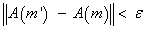holds. By continuity of f and compactness of M the function f is uniformly continuous, so there is a positive number δ such that if u, v ∈ M and ||u − v || < δ, then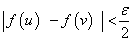. By Archimedean property of numbers, there is a natural number k for which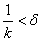. For each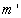in the ball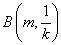and each s ∈ S we have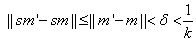because the action is non-expansive. Now use uniform continuous property of f to get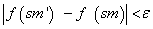. Hence corresponds to ε>0 we found the ball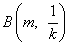so that if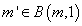, then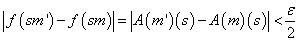for all s ∈ S. Consequently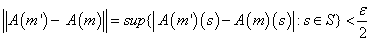which shows that A is continuous. On the other hand for each right translate of fm=A(m) we have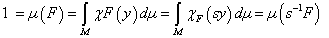that is raA(m)=A(am) hence {rafm : a ∈ S}=A(Sm). The set Sm is relatively compact in M and A is continuous, so A(Sm) is relatively compact in the sup-norm topology of C(S). Therefore by theorem 2.1 part (a) we see that fm= A(m) ∈ AP(S) and from part (b) fm ∈ LU C(S).

Now we use the above lemma to modify Takahashi’s proof  for left amenable semi- topological semigroups which are not necessarily discrete.

Theorem 2.3: Let K be a non-empty compact convex subset of a Banach space E and S be a left amenable semi-topological semigroup which acts on K separately continuous and non-expansive. Then S has a common fixed point in K.

Proof: An application of Zorn’s lemma shows that there exists a minimal non-empty compact convex and S-invariant subset X ⊆ K. If X is a singleton we are done, otherwise apply Zorn’s lemma for the second time to get a minimal non-empty compact and S- invariant subset M ⊆ X.

We claim that M is S-preserved, i.e. M=sM for all s ∈ S. Let ν be a left invariant mean on LUC (S) and define μ(f)=ν(fm), where fm is defined as in lemma 2.2. Then by Riesz representation theorem, μ induces a regular probability measure on M (still denoted by μ) such that μ(sB)=μ(B) for all Borel sets B ⊆ M and s ∈ S. Let F be the support of μ. Each s ∈ S defines a measurable continuous function from M into M, so by basic properties of support F ⊆ sM, μ(sM)=μ(M)=1 . Assume that χF is the characteristic function of F. For each s ∈ S,(s−1F means the pre-image of F under s) again by the definition and properties of support we see that F ⊆s−1F , meaning that F is S-invariant. Hence F=M by the minimality of M. Consequently M=F ⊆ ⊆ sM for each s ∈ S. But M was already S-invariant, so sM=M for each s in S.

Now if M is singleton we are done, otherwise if δ(M)=diam(M) >0, we get a contradiction by DeMarr’s lemma  which implies that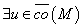such that r0=sup{||m – u||: m ∈ M } < δ(M).

Define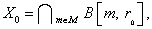then X0 is a non-empty (indeed u ∈ X0) compact convex proper subset of X such that sX0 ⊆ X0 for each s in S (the inclusion follows from the fact that M is S-preserved). But this contradicts the minimality of X. Therefore M contains only one point which is a common fixed point for the action of S.

Obviously every amenable discrete semigroup is a left amenable semi-topological semi- group, so we can deduce Takahashi’s theorem from our theorem:

Corollary 2.4: Let K is a non-empty compact convex subset of a Banach space E and S is an amenable discrete semigroup which acts on K separately continuous and non-expansive. Then S has a common fixed point in K .

#### References

Select your language of interest to view the total content in your interested language

### Article Usage

• Total views: 12456
• [From(publication date):
November-2015 - Jan 24, 2020]
• Breakdown by view type
• HTML page views : 8550Can't read the image? click here to refresh